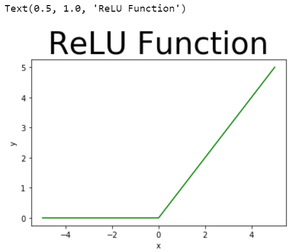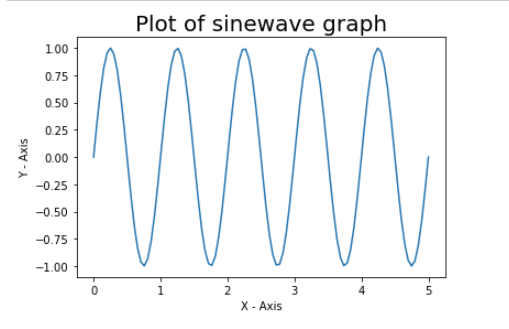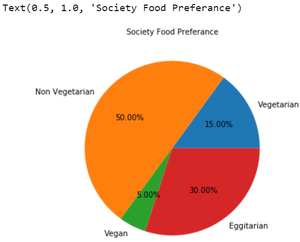# How to change the font size of the Title in a Matplotlib figure ?

In this article, we are going to discuss how to change the font size of the title in a figure using matplotlib module in Python.

As we use matplotlib.pyplot.title() method to assign a title to a plot, so in order to change the font size, we are going to use the font size argument of the pyplot.title() method in the matplotlib module.

### Example 1: Change the font size of the Title in a Matplotlib

In this example, we are plotting a ReLU function graph with fontsize=40.

## Python3

 `# importing module` `import` `matplotlib.pyplot as plt`   `# assigning x and y coordinates` `x ``=` `[``-``5``, ``-``4``, ``-``3``, ``-``2``, ``-``1``, ``0``, ``1``, ``2``, ``3``, ``4``, ``5``]` `y ``=` `[]`   `for` `i ``in` `range``(``len``(x)):` `    ``y.append(``max``(``0``, x[i]))`   `# depicting the visualization` `plt.plot(x, y, color``=``'green'``)` `plt.xlabel(``'x'``)` `plt.ylabel(``'y'``)`   `# displaying the title` `plt.title(``"ReLU Function"``,` `          ``fontsize ``=` `40``)`

Output:### Example 2: Set the figure title font size in matplotlib

In this example, we are plotting a sinewave graph with set_size(20).

## Python3

 `import` `numpy as np` `import` `matplotlib.pyplot as plt`   `xaxis``=``np.linspace(``0``,``5``,``100``)` `yaxis``=` `np.sin(``2` `*` `np.pi ``*` `x)`   `axes ``=` `plt.gca()` `plt.plot(xaxis, yaxis)` `axes.set_title(``'Plot of sinwave graph'``)` `axes.set_xlabel(``'X - Axis'``)` `axes.set_ylabel(``'Y - Axis'``)`   `axes.title.set_size(``20``)`   `plt.show()`

Output:### Example 3: Set the figure title font size in matplotlib

In this example, we are plotting a pie graph with fontsize=10.

## Python3

 `# importing modules` `from` `matplotlib ``import` `pyplot as plt`   `# assigning x and y coordinates` `foodPreference ``=` `[``'Vegetarian'``, ``'Non Vegetarian'``,` `                                ``'Vegan'``, ``'Eggitarian'``]`   `consumers ``=` `[``30``, ``100``, ``10``, ``60``]`   `# depicting the visualization` `fig ``=` `plt.figure()` `ax ``=` `fig.add_axes([``0``, ``0``, ``1``, ``1``])` `ax.axis(``'equal'``)` `ax.pie(consumers, labels ``=` `foodPreference,` `       ``autopct``=``'%1.2f%%'``)`   `# displaying the title` `plt.title(``"Society Food Preference"``,` `          ``fontsize ``=` `10``)`

Output:Whether you're preparing for your first job interview or aiming to upskill in this ever-evolving tech landscape, GeeksforGeeks Courses are your key to success. We provide top-quality content at affordable prices, all geared towards accelerating your growth in a time-bound manner. Join the millions we've already empowered, and we're here to do the same for you. Don't miss out - check it out now!